library: libGeom#include "TGeoManager.h"

# TGeoManager

class description - source file - inheritance tree (.pdf)

## class TGeoManager : public TNamed

Inheritance Chart:
 TObject
<-
TNamed
<-
TGeoManager
```    private:
void BuildCache(Bool_t dummy = kFALSE, Bool_t nodeid = kFALSE)
void BuildIdArray()
TGeoNode* FindInCluster(Int_t* cluster, Int_t nc)
Int_t GetTouchedCluster(Int_t start, Double_t* point, Int_t* check_list, Int_t ncheck, Int_t* result)
void Init()
Bool_t IsLoopingVolumes() const
void SafetyOverlaps()
void SetLoopVolumes(Bool_t flag = kTRUE)
void Voxelize(Option_t* option = "0")

public:
TGeoManager()
TGeoManager(const char* name, const char* title)
TGeoManager(const TGeoManager&)
virtual ~TGeoManager()
Int_t AddTrack(Int_t id, Int_t pdgcode, TObject* particle = 0)
void AnimateTracks(Double_t tmin = 0, Double_t tmax = 5E-8, Int_t nframes = 200, Option_t* option = "/*")
void BombTranslation(const Double_t* tr, Double_t* bombtr)
virtual void Browse(TBrowser* b)
void BuildDefaultMaterials()
virtual Bool_t cd(const char* path)
void CdDown(Int_t index)
void CdNode(Int_t nodeid)
void CdTop()
void CdUp()
void CheckGeometry(Option_t* option)
void CheckOverlaps(Double_t ovlp = 0.1, Option_t* option)
void CheckPoint(Double_t x = 0, Double_t y = 0, Double_t z = 0, Option_t* option)
static TClass* Class()
void CleanGarbage()
void ClearAttributes()
void ClearOverlaps()
void ClearPhysicalNodes(Bool_t mustdelete = kFALSE)
void ClearShape(const TGeoShape* shape)
void ClearTracks()
void CloseGeometry(Option_t* option = "d")
Int_t CountNodes(const TGeoVolume* vol = 0, Int_t nlevels = 1000, Int_t option = 0)
void DefaultAngles()
void DefaultColors()
TGeoVolume* Division(const char* name, const char* mother, Int_t iaxis, Int_t ndiv, Double_t start, Double_t step, Int_t numed = 0, Option_t* option)
void DrawCurrentPoint(Int_t color = 2)
void DrawPath(const char* path)
void DrawTracks(Option_t* option)
virtual Int_t Export(const char* filename, const char* name, Option_t* option = "v")
TGeoMaterial* FindDuplicateMaterial(const TGeoMaterial* mat) const
TGeoNode* FindNextBoundary(Double_t stepmax = 1e30, const char* path)
TGeoNode* FindNode(Bool_t safe_start = kTRUE)
TGeoNode* FindNode(Double_t x, Double_t y, Double_t z)
Double_t* FindNormal(Bool_t forward = kTRUE)
Double_t* FindNormalFast()
UChar_t* GetBits()
void GetBombFactors(Double_t& bombx, Double_t& bomby, Double_t& bombz, Double_t& bombr) const
Int_t GetBombMode() const
void GetBranchNames(Int_t* names) const
void GetBranchNumbers(Int_t* copyNumbers, Int_t* volumeNumbers) const
void GetBranchOnlys(Int_t* isonly) const
virtual Int_t GetByteCount(Option_t* option = "0")
TGeoNodeCache* GetCache() const
Double_t* GetCldir() const
Double_t* GetCldirChecked() const
TGeoShape* GetClippingShape() const
Double_t* GetCurrentDirection() const
TGeoHMatrix* GetCurrentMatrix() const
TGeoNode* GetCurrentNode() const
Int_t GetCurrentNodeId() const
Double_t* GetCurrentPoint() const
TVirtualGeoTrack* GetCurrentTrack()
TGeoVolume* GetCurrentVolume() const
Double_t* GetDblBuffer(Int_t length)
TVirtualGeoPainter* GetGeomPainter()
TGeoHMatrix* GetGLMatrix() const
TGeoHMatrix* GetHMatrix()
Int_t* GetIntBuffer(Int_t length)
const Double_t* GetLastPoint() const
TVirtualGeoTrack* GetLastTrack()
Int_t GetLevel() const
TObjArray* GetListOfGVolumes() const
TList* GetListOfMaterials() const
TObjArray* GetListOfMatrices() const
TList* GetListOfMedia() const
TObjArray* GetListOfNodes()
TObjArray* GetListOfOverlaps()
TObjArray* GetListOfPhysicalNodes()
TObjArray* GetListOfShapes() const
TObjArray* GetListOfTracks() const
TObjArray* GetListOfUVolumes() const
TObjArray* GetListOfVolumes() const
TGeoVolume* GetMasterVolume() const
TGeoMaterial* GetMaterial(const char* matname) const
TGeoMaterial* GetMaterial(Int_t id) const
Int_t GetMaterialIndex(const char* matname) const
Int_t GetMaxVisNodes() const
TGeoMedium* GetMedium(const char* medium) const
TGeoMedium* GetMedium(Int_t numed) const
TGeoNode* GetMother(Int_t up = 1) const
TGeoNode* GetNextNode() const
Int_t GetNNodes()
TGeoNode* GetNode(Int_t level) const
Int_t GetNodeId() const
const Double_t* GetNormal() const
Int_t GetNsegments() const
Int_t GetNtracks() const
TVirtualGeoPainter* GetPainter() const
TGeoVolume* GetPaintVolume() const
TVirtualGeoTrack* GetParentTrackOfId(Int_t id) const
const char* GetParticleName() const
const char* GetPath() const
const char* GetPdgName(Int_t pdg) const
TGeoPhysicalNode* GetPhysicalNode(Int_t i) const
Double_t GetSafeDistance() const
Int_t GetSafeLevel() const
Int_t GetStackLevel() const
Double_t GetStep() const
Double_t GetTmax() const
Bool_t GetTminTmax(Double_t& tmin, Double_t& tmax) const
TGeoNode* GetTopNode() const
TGeoVolume* GetTopVolume() const
TVirtualGeoTrack* GetTrack(Int_t index)
Int_t GetTrackIndex(Int_t id) const
TVirtualGeoTrack* GetTrackOfId(Int_t id) const
Int_t GetUID(const char* volname) const
Int_t GetVirtualLevel()
Double_t GetVisDensity() const
Int_t GetVisLevel() const
Int_t GetVisOption() const
TGeoVolume* GetVolume(const char* name) const
TGeoVolume* GetVolume(Int_t uid) const
Bool_t GotoSafeLevel()
static TGeoManager* Import(const char* filename, const char* name, Option_t* option)
TGeoNode* InitTrack(Double_t* point, Double_t* dir)
TGeoNode* InitTrack(Double_t x, Double_t y, Double_t z, Double_t nx, Double_t ny, Double_t nz)
virtual TClass* IsA() const
Bool_t IsAnimatingTracks() const
Bool_t IsCheckingOverlaps() const
Bool_t IsClosed() const
Bool_t IsCurrentOverlapping() const
Bool_t IsDrawingExtra() const
Bool_t IsEntering() const
Bool_t IsExiting() const
virtual Bool_t IsFolder() const
Bool_t IsInPhiRange() const
Bool_t IsMatrixReflection() const
Bool_t IsMatrixTransform() const
Bool_t IsNullStep() const
Bool_t IsOnBoundary() const
Bool_t IsOutside() const
Bool_t IsSameLocation(Double_t x, Double_t y, Double_t z, Bool_t change = kFALSE)
Bool_t IsSameLocation() const
Bool_t IsSamePoint(Double_t x, Double_t y, Double_t z) const
Bool_t IsStartSafe() const
Bool_t IsStepEntering() const
Bool_t IsStepExiting() const
Bool_t IsStreamingVoxels() const
Bool_t IsVisLeaves() const
void LocalToMaster(const Double_t* local, Double_t* master) const
void LocalToMasterBomb(const Double_t* local, Double_t* master) const
void LocalToMasterVect(const Double_t* local, Double_t* master) const
TGeoVolume* MakeArb8(const char* name, const TGeoMedium* medium, Double_t dz, Double_t* vertices = 0)
TGeoVolume* MakeBox(const char* name, const TGeoMedium* medium, Double_t dx, Double_t dy, Double_t dz)
TGeoVolume* MakeCone(const char* name, const TGeoMedium* medium, Double_t dz, Double_t rmin1, Double_t rmax1, Double_t rmin2, Double_t rmax2)
TGeoVolume* MakeCons(const char* name, const TGeoMedium* medium, Double_t dz, Double_t rmin1, Double_t rmax1, Double_t rmin2, Double_t rmax2, Double_t phi1, Double_t phi2)
TGeoVolume* MakeCtub(const char* name, const TGeoMedium* medium, Double_t rmin, Double_t rmax, Double_t dz, Double_t phi1, Double_t phi2, Double_t lx, Double_t ly, Double_t lz, Double_t tx, Double_t ty, Double_t tz)
TGeoVolume* MakeEltu(const char* name, const TGeoMedium* medium, Double_t a, Double_t b, Double_t dz)
TGeoVolume* MakeGtra(const char* name, const TGeoMedium* medium, Double_t dz, Double_t theta, Double_t phi, Double_t twist, Double_t h1, Double_t bl1, Double_t tl1, Double_t alpha1, Double_t h2, Double_t bl2, Double_t tl2, Double_t alpha2)
TGeoVolume* MakeHype(const char* name, const TGeoMedium* medium, Double_t rin, Double_t stin, Double_t rout, Double_t stout, Double_t dz)
TGeoVolume* MakePara(const char* name, const TGeoMedium* medium, Double_t dx, Double_t dy, Double_t dz, Double_t alpha, Double_t theta, Double_t phi)
TGeoVolume* MakeParaboloid(const char* name, const TGeoMedium* medium, Double_t rlo, Double_t rhi, Double_t dz)
TGeoVolume* MakePcon(const char* name, const TGeoMedium* medium, Double_t phi, Double_t dphi, Int_t nz)
TGeoVolume* MakePgon(const char* name, const TGeoMedium* medium, Double_t phi, Double_t dphi, Int_t nedges, Int_t nz)
TGeoPhysicalNode* MakePhysicalNode(const char* path = "0")
TGeoVolume* MakeSphere(const char* name, const TGeoMedium* medium, Double_t rmin, Double_t rmax, Double_t themin = 0, Double_t themax = 180, Double_t phimin = 0, Double_t phimax = 360)
TGeoVolume* MakeTorus(const char* name, const TGeoMedium* medium, Double_t r, Double_t rmin, Double_t rmax, Double_t phi1 = 0, Double_t dphi = 360)
TVirtualGeoTrack* MakeTrack(Int_t id, Int_t pdgcode, TObject* particle)
TGeoVolume* MakeTrap(const char* name, const TGeoMedium* medium, Double_t dz, Double_t theta, Double_t phi, Double_t h1, Double_t bl1, Double_t tl1, Double_t alpha1, Double_t h2, Double_t bl2, Double_t tl2, Double_t alpha2)
TGeoVolume* MakeTrd1(const char* name, const TGeoMedium* medium, Double_t dx1, Double_t dx2, Double_t dy, Double_t dz)
TGeoVolume* MakeTrd2(const char* name, const TGeoMedium* medium, Double_t dx1, Double_t dx2, Double_t dy1, Double_t dy2, Double_t dz)
TGeoVolume* MakeTube(const char* name, const TGeoMedium* medium, Double_t rmin, Double_t rmax, Double_t dz)
TGeoVolume* MakeTubs(const char* name, const TGeoMedium* medium, Double_t rmin, Double_t rmax, Double_t dz, Double_t phi1, Double_t phi2)
TGeoVolumeAssembly* MakeVolumeAssembly(const char* name)
TGeoVolumeMulti* MakeVolumeMulti(const char* name, const TGeoMedium* medium)
TGeoVolume* MakeXtru(const char* name, const TGeoMedium* medium, Int_t nz)
void MasterToLocal(const Double_t* master, Double_t* local) const
void MasterToLocalBomb(const Double_t* master, Double_t* local) const
void MasterToLocalVect(const Double_t* master, Double_t* local) const
void MasterToTop(const Double_t* master, Double_t* top) const
TGeoMaterial* Material(const char* name, Double_t a, Double_t z, Double_t dens, Int_t uid, Double_t radlen = 0, Double_t intlen = 0)
void Matrix(Int_t index, Double_t theta1, Double_t phi1, Double_t theta2, Double_t phi2, Double_t theta3, Double_t phi3)
TGeoMedium* Medium(const char* name, Int_t numed, Int_t nmat, Int_t isvol, Int_t ifield, Double_t fieldm, Double_t tmaxfd, Double_t stemax, Double_t deemax, Double_t epsil, Double_t stmin)
TGeoMaterial* Mixture(const char* name, Float_t* a, Float_t* z, Double_t dens, Int_t nelem, Float_t* wmat, Int_t uid)
TGeoMaterial* Mixture(const char* name, Double_t* a, Double_t* z, Double_t dens, Int_t nelem, Double_t* wmat, Int_t uid)
void Node(const char* name, Int_t nr, const char* mother, Double_t x, Double_t y, Double_t z, Int_t irot, Bool_t isOnly, Float_t* upar, Int_t npar = 0)
void Node(const char* name, Int_t nr, const char* mother, Double_t x, Double_t y, Double_t z, Int_t irot, Bool_t isOnly, Double_t* upar, Int_t npar = 0)
TGeoManager& operator=(const TGeoManager&)
void OptimizeVoxels(const char* filename = "tgeovox.C")
static Int_t Parse(const char* expr, TString& expr1, TString& expr2, TString& expr3)
void PopDummy(Int_t ipop = 9999)
Bool_t PopPath()
Bool_t PopPath(Int_t index)
Bool_t PopPoint()
Bool_t PopPoint(Int_t index)
void PrintOverlaps() const
Int_t PushPath(Int_t startlevel = 0)
Int_t PushPoint(Int_t startlevel = 0)
void RandomPoints(const TGeoVolume* vol, Int_t npoints = 10000, Option_t* option)
void RandomRays(Int_t nrays = 1000, Double_t startx = 0, Double_t starty = 0, Double_t startz = 0)
void RegisterMatrix(const TGeoMatrix* matrix)
void RemoveMaterial(Int_t index)
void ResetUserData()
void RestoreMasterVolume()
Double_t Safety(Bool_t inside = kFALSE)
TGeoNode* SamplePoints(Int_t npoints, Double_t& dist, Double_t epsil = 1E-5, const char* g3path)
void SaveAttributes(const char* filename = "tgeoatt.C")
TGeoNode* SearchNode(Bool_t downwards = kFALSE, const TGeoNode* skipnode = 0)
void SelectTrackingMedia()
void SetAnimateTracks(Bool_t flag = kTRUE)
void SetBombFactors(Double_t bombx = 1.3, Double_t bomby = 1.3, Double_t bombz = 1.3, Double_t bombr = 1.3)
void SetCache(const TGeoNodeCache* cache)
void SetCldirChecked(Double_t* dir)
void SetClipping(Bool_t flag = kTRUE)
void SetClippingShape(TGeoShape* clip)
void SetCurrentDirection(Double_t* dir)
void SetCurrentDirection(Double_t nx, Double_t ny, Double_t nz)
void SetCurrentPoint(Double_t* point)
void SetCurrentPoint(Double_t x, Double_t y, Double_t z)
void SetCurrentTrack(Int_t i)
void SetCurrentTrack(TVirtualGeoTrack* track)
void SetDrawExtraPaths(Bool_t flag = kTRUE)
void SetExplodedView(Int_t iopt = 0)
void SetLastPoint(Double_t x, Double_t y, Double_t z)
void SetMatrixReflection(Bool_t flag = kTRUE)
void SetMatrixTransform(Bool_t on = kTRUE)
void SetMaxVisNodes(Int_t maxnodes = 10000)
void SetNsegments(Int_t nseg)
void SetOutside(Bool_t flag = kTRUE)
void SetPaintVolume(TGeoVolume* vol)
void SetParticleName(const char* pname)
void SetPdgName(Int_t pdg, const char* name)
void SetPhiRange(Double_t phimin = 0., Double_t phimax = 360.)
void SetStartSafe(Bool_t flag = kTRUE)
void SetStep(Double_t step)
void SetTminTmax(Double_t tmin = 0, Double_t tmax = 999)
void SetTopVisible(Bool_t vis = kTRUE)
void SetTopVolume(TGeoVolume* vol)
void SetVisDensity(Double_t dens = 0.01)
void SetVisLevel(Int_t level = 3)
void SetVisOption(Int_t option = 0)
void SetVolumeAttribute(const char* name, const char* att, Int_t val)
virtual void ShowMembers(TMemberInspector& insp, char* parent)
virtual ULong_t SizeOf(const TGeoNode* node, Option_t* option)
void SortOverlaps()
TGeoNode* Step(Bool_t is_geom = kTRUE, Bool_t cross = kTRUE)
virtual void Streamer(TBuffer& b)
void StreamerNVirtual(TBuffer& b)
void Test(Int_t npoints = 1000000, Option_t* option)
void TestOverlaps(const char* path)
void TopToMaster(const Double_t* top, Double_t* master) const
void UnbombTranslation(const Double_t* tr, Double_t* bombtr)
void UpdateCurrentPosition(Double_t* nextpoint)
void ViewLeaves(Bool_t flag = kTRUE)
TGeoVolume* Volume(const char* name, const char* shape, Int_t nmed, Float_t* upar, Int_t npar = 0)
TGeoVolume* Volume(const char* name, const char* shape, Int_t nmed, Double_t* upar, Int_t npar = 0)
Double_t Weight(Double_t precision = 0.01, Option_t* option = "v")
```

### Data Members

```    private:
Double_t fStep                ! step to be done from current point and direction
Double_t fSafety              ! safety radius from current point
Double_t fLastSafety          ! last computed safety radius
Double_t fPhimin              ! lowest range for phi cut
Double_t fPhimax              ! highest range for phi cut
Double_t fTmin                ! lower time limit for tracks drawing
Double_t fTmax                ! upper time limit for tracks drawing
Int_t fLevel               ! current geometry level;
Int_t fNNodes              total number of physical nodes
TString fPath                ! path to current node
TString fParticleName        ! particles to be drawn
Double_t fNormal           ! cosine of incident angle on current checked surface
Double_t* fCldir               ! unit vector to current closest shape
Double_t* fCldirChecked        ! unit vector to current checked shape
Double_t* fPoint               ! current point
Double_t* fDirection           ! current direction
Double_t fVisDensity          transparency threshold by density
Int_t fExplodedView        exploded view mode
Int_t fVisOption           global visualization option
Int_t fVisLevel            maximum visualization depth
Int_t fNsegments           number of segments to approximate circles
Int_t fNtracks             number of tracks
Int_t fMaxVisNodes         maximum number of visible nodes
TVirtualGeoTrack* fCurrentTrack        ! current track
Int_t fNpdg                number of different pdg's stored
Int_t fPdgId          pdg conversion table
Bool_t fSearchOverlaps      ! flag set when an overlapping cluster is searched
Bool_t fCurrentOverlapping  ! flags the type of the current node
Bool_t fLoopVolumes         ! flag volume lists loop
Bool_t fStartSafe           ! flag a safe start for point classification
Bool_t fIsEntering          ! flag if current step just got into a new node
Bool_t fIsExiting           ! flag that current track is about to leave current node
Bool_t fIsStepEntering      ! flag that next geometric step will enter new volume
Bool_t fIsStepExiting       ! flaag that next geometric step will exit current volume
Bool_t fIsOutside           ! flag that current point is outside geometry
Bool_t fIsOnBoundary        ! flag that current point is on some boundary
Bool_t fIsSameLocation      ! flag that a new point is in the same node as previous
Bool_t fIsNullStep          ! flag that last geometric step was null
Bool_t fStreamVoxels        flag to allow voxelization I/O
Bool_t fPhiCut              flag for phi cuts
Bool_t fTimeCut             time cut for tracks
Bool_t fDrawExtra           ! flag that the list of physical nodes has to be drawn
Bool_t fMatrixTransform     ! flag for using GL matrix
Bool_t fMatrixReflection    ! flag for GL reflections
TGeoNodeCache* fCache               ! cache for physical nodes
TVirtualGeoPainter* fPainter             ! current painter
TObjArray* fMatrices            -> list of local transformations
TObjArray* fShapes              -> list of shapes
TObjArray* fVolumes             -> list of volumes
TObjArray* fPhysicalNodes       -> list of physical nodes
TObjArray* fGShapes             ! list of runtime shapes
TObjArray* fGVolumes            ! list of runtime volumes
TObjArray* fTracks              -> list of tracks attached to geometry
TObjArray* fPdgNames            -> list of pdg names for tracks
TList* fMaterials           -> list of materials
TList* fMedia               -> list of tracking media
TObjArray* fNodes               -> current branch of nodes
TObjArray* fOverlaps            -> list of geometrical overlaps
UChar_t* fBits                ! bits used for voxelization
TGeoVolume* fCurrentVolume       ! current volume
TGeoVolume* fTopVolume           ! top level volume in geometry
TGeoNode* fCurrentNode         ! current node
TGeoNode* fTopNode             ! top physical node
TGeoNode* fLastNode            ! last searched node
TGeoNode* fNextNode            ! next node that will be crossed
TGeoVolume* fMasterVolume        master volume
TGeoHMatrix* fCurrentMatrix       ! current global matrix
TGeoHMatrix* fGLMatrix            matrix to be used for view transformations
TObjArray* fUniqueVolumes       -> list of unique volumes
TGeoShape* fClippingShape       ! clipping shape for raytracing
Int_t* fNodeIdArray         ! array of node id's
Int_t fIntSize             ! int buffer size
Int_t fDblSize             ! dbl buffer size
Int_t fOverlapSize         ! current size of fOverlapClusters
Int_t fOverlapMark         ! current recursive position in fOverlapClusters
Int_t* fIntBuffer           ! transient int buffer
Int_t* fOverlapClusters     ! internal array for overlaps
Double_t* fDblBuffer           ! transient dbl buffer
Double_t fLastPoint        ! last point for which safety was computed
TGeoVolume* fPaintVolume         ! volume currently painted
```

## Class Description

``` General architecture
--------------------

The new ROOT geometry package is a tool designed for building, browsing,
tracking and visualizing a detector geometry. The code is independent from
other external MC for simulation, therefore it does not contain any
constraints related to physics. However, the package defines a number of
hooks for tracking, such as media, materials, magnetic field or track state flags,
in order to allow interfacing to tracking MC's. The final goal is to be
able to use the same geometry for several purposes, such as tracking,
reconstruction or visualization, taking advantage of the ROOT features
related to bookkeeping, I/O, histograming, browsing and GUI's.

The geometrical modeler is the most important component of the package and
it provides answers to the basic questions like "Where am I ?" or "How far
from the next boundary ?", but also to more complex ones like "How far from
the closest surface ?" or "Which is the next crossing along a helix ?".

The architecture of the modeler is a combination between a GEANT-like
containment scheme and a normal CSG binary tree at the level of shapes. An
important common feature of all detector geometry descriptions is the
mother-daughter concept. This is the most natural approach when tracking
is concerned and imposes a set of constraints to the way geometry is defined.
Constructive solid geometry composition is used only in order to create more
complex shapes from an existing set of primitives through boolean operations.
This feature is not implemented yet but in future full definition of boolean
expressions will be supported.

Practically every geometry defined in GEANT style can be mapped by the modeler.
The basic components used for building the logical hierarchy of the geometry
are called "volumes" and "nodes". Volumes (sometimes called "solids") are fully
defined geometrical objects having a given shape and medium and possibly
containing a list of nodes. Nodes represent just positioned instances of volumes
inside a container volume and they are not directly defined by user. They are
automatically created as a result of adding one volume inside other or dividing
a volume. The geometrical transformation hold by nodes is always defined with
respect to their mother (relative positioning). Reflection matrices are allowed.
All volumes have to be fully aware of their containees when the geometry is
closed. They will build aditional structures (voxels) in order to fasten-up
the search algorithms. Finally, nodes can be regarded as bidirectional links
between containers and containees objects.

The structure defined in this way is a graph structure since volumes are
replicable (same volume can become daughter node of several other volumes),
every volume becoming a branch in this graph. Any volume in the logical graph
can become the actual top volume at run time (see TGeoManager::SetTopVolume()).
All functionalities of the modeler will behave in this case as if only the
corresponding branch starting from this volume is the registered geometry.

```
/*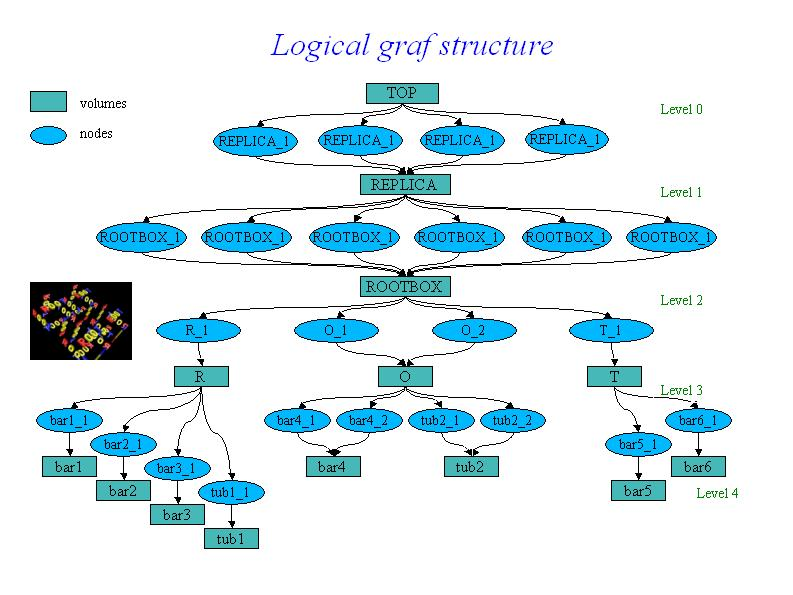*/
```
A given volume can be positioned several times in the geometry. A volume
can be divided according default or user-defined patterns, creating automatically
the list of division nodes inside. The elementary volumes created during the
dividing process follow the same scheme as usual volumes, therefore it is possible
to position further geometrical structures inside or to divide them further more
(see TGeoVolume::Divide()).

The primitive shapes supported by the package are basically the GEANT3
shapes (see class TGeoShape), arbitrary wedges with eight vertices on two parallel
planes. All basic primitives inherits from class TGeoBBox since the bounding box
of a solid is essential for the tracking algorithms. They also implement the
virtual methods defined in the virtual class TGeoShape (point and segment
classification). User-defined primitives can be direcly plugged into the modeler
provided that they override these methods. Composite shapes will be soon supported
by the modeler. In order to build a TGeoCompositeShape, one will have to define
first the primitive components. The object that handle boolean
operations among components is called TGeoBoolCombinator and it has to be
constructed providing a string boolean expression between the components names.

Example for building a simple geometry :
-----------------------------------------

______________________________________________________________________________
void rootgeom()
{
//--- Definition of a simple geometry
TGeoManager *geom = new TGeoManager("simple1", "Simple geometry");

//--- define some materials
TGeoMaterial *matVacuum = new TGeoMaterial("Vacuum", 0,0,0);
TGeoMaterial *matAl = new TGeoMaterial("Al", 26.98,13,2.7);
//--- define some media
TGeoMedium *med;
TGeoMedium *Vacuum = new TGeoMedium(1, matVacuum);
TGeoMedium *Al = new TGeoMedium(2, matAl);

//--- define the transformations
TGeoTranslation *tr1 = new TGeoTranslation(20., 0, 0.);
TGeoTranslation *tr2 = new TGeoTranslation(10., 0., 0.);
TGeoTranslation *tr3 = new TGeoTranslation(10., 20., 0.);
TGeoTranslation *tr4 = new TGeoTranslation(5., 10., 0.);
TGeoTranslation *tr5 = new TGeoTranslation(20., 0., 0.);
TGeoTranslation *tr6 = new TGeoTranslation(-5., 0., 0.);
TGeoTranslation *tr7 = new TGeoTranslation(7.5, 7.5, 0.);
TGeoRotation   *rot1 = new TGeoRotation("rot1", 90., 0., 90., 270., 0., 0.);
TGeoCombiTrans *combi1 = new TGeoCombiTrans(7.5, -7.5, 0., rot1);
TGeoTranslation *tr8 = new TGeoTranslation(7.5, -5., 0.);
TGeoTranslation *tr9 = new TGeoTranslation(7.5, 20., 0.);
TGeoTranslation *tr10 = new TGeoTranslation(85., 0., 0.);
TGeoTranslation *tr11 = new TGeoTranslation(35., 0., 0.);
TGeoTranslation *tr12 = new TGeoTranslation(-15., 0., 0.);
TGeoTranslation *tr13 = new TGeoTranslation(-65., 0., 0.);

TGeoTranslation  *tr14 = new TGeoTranslation(0,0,-100);
TGeoCombiTrans *combi2 = new TGeoCombiTrans(0,0,100,
new TGeoRotation("rot2",90,180,90,90,180,0));
TGeoCombiTrans *combi3 = new TGeoCombiTrans(100,0,0,
new TGeoRotation("rot3",90,270,0,0,90,180));
TGeoCombiTrans *combi4 = new TGeoCombiTrans(-100,0,0,
new TGeoRotation("rot4",90,90,0,0,90,0));
TGeoCombiTrans *combi5 = new TGeoCombiTrans(0,100,0,
new TGeoRotation("rot5",0,0,90,180,90,270));
TGeoCombiTrans *combi6 = new TGeoCombiTrans(0,-100,0,
new TGeoRotation("rot6",180,0,90,180,90,90));

//--- make the top container volume
Double_t worldx = 110.;
Double_t worldy = 50.;
Double_t worldz = 5.;
TGeoVolume *top = geom->MakeBox("TOP", Vacuum, 270., 270., 120.);
geom->SetTopVolume(top); // mandatory !
//--- build other container volumes
TGeoVolume *replica = geom->MakeBox("REPLICA", Vacuum,120,120,120);
replica->SetVisibility(kFALSE);
TGeoVolume *rootbox = geom->MakeBox("ROOT", Vacuum, 110., 50., 5.);
rootbox->SetVisibility(kFALSE); // this will hold word 'ROOT'

//--- make letter 'R'
TGeoVolume *R = geom->MakeBox("R", Vacuum, 25., 25., 5.);
R->SetVisibility(kFALSE);
TGeoVolume *bar1 = geom->MakeBox("bar1", Al, 5., 25, 5.);
bar1->SetLineColor(kRed);
TGeoVolume *bar2 = geom->MakeBox("bar2", Al, 5., 5., 5.);
bar2->SetLineColor(kRed);
TGeoVolume *tub1 = geom->MakeTubs("tub1", Al, 5., 15., 5., 90., 270.);
tub1->SetLineColor(kRed);
TGeoVolume *bar3 = geom->MakeArb8("bar3", Al, 5.);
bar3->SetLineColor(kRed);
TGeoArb8 *arb = (TGeoArb8*)bar3->GetShape();
arb->SetVertex(0, 15., -5.);
arb->SetVertex(1, 5., -5.);
arb->SetVertex(2, -10., -25.);
arb->SetVertex(3, 0., -25.);
arb->SetVertex(4, 15., -5.);
arb->SetVertex(5, 5., -5.);
arb->SetVertex(6, -10., -25.);
arb->SetVertex(7, 0., -25.);

//--- make letter 'O'
TGeoVolume *O = geom->MakeBox("O", Vacuum, 25., 25., 5.);
O->SetVisibility(kFALSE);
TGeoVolume *bar4 = geom->MakeBox("bar4", Al, 5., 7.5, 5.);
bar4->SetLineColor(kYellow);
TGeoVolume *tub2 = geom->MakeTubs("tub1", Al, 7.5, 17.5, 5., 0., 180.);
tub2->SetLineColor(kYellow);

//--- make letter 'T'
TGeoVolume *T = geom->MakeBox("T", Vacuum, 25., 25., 5.);
T->SetVisibility(kFALSE);
TGeoVolume *bar5 = geom->MakeBox("bar5", Al, 5., 20., 5.);
bar5->SetLineColor(kBlue);
TGeoVolume *bar6 = geom->MakeBox("bar6", Al, 17.5, 5., 5.);
bar6->SetLineColor(kBlue);

//--- add letters to 'ROOT' container

//--- add word 'ROOT' on each face of a cube

//--- add four replicas of this cube to top volume
top->AddNode(replica, 1, new TGeoTranslation(-150, -150, 0));
top->AddNode(replica, 2, new TGeoTranslation(150, -150, 0));
top->AddNode(replica, 3, new TGeoTranslation(150, 150, 0));
top->AddNode(replica, 4, new TGeoTranslation(-150, 150, 0));

//--- close the geometry
geom->CloseGeometry();

//--- draw the ROOT box
geom->SetVisLevel(4);
top->Draw();
}
______________________________________________________________________________

```
/*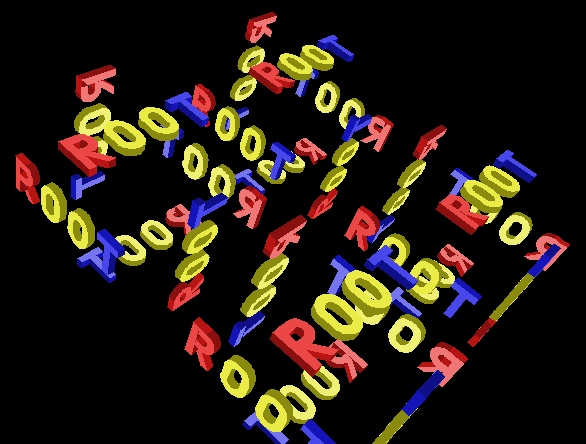*/
```

TGeoManager - the manager class for the geometry package.
---------------------------------------------------------

TGeoManager class is embedding all the API needed for building and tracking
a geometry. It defines a global pointer (gGeoManager) in order to be fully
accessible from external code. The mechanism of handling multiple geometries
at the same time will be soon implemented.

TGeoManager is the owner of all geometry objects defined in a session,
therefore users must not try to control their deletion. It contains lists of
media, materials, transformations, shapes and volumes. Logical nodes (positioned
volumes) are created and destroyed by the TGeoVolume class. Physical
nodes and their global transformations are subjected to a caching mechanism
due to the sometimes very large memory requirements of logical graph expansion.
The caching mechanism is triggered by the total number of physical instances
of volumes and the cache manager is a client of TGeoManager. The manager class
also controls the painter client. This is linked with ROOT graphical libraries
loaded on demand in order to control visualization actions.

Rules for building a valid geometry
-----------------------------------

A given geometry can be built in various ways, but there are mandatory steps
that have to be followed in order to be validated by the modeler. There are
general rules : volumes needs media and shapes in order to be created,
both container an containee volumes must be created before linking them together,
and the relative transformation matrix must be provided. All branches must
have an upper link point otherwise they will not be considered as part of the
geometry. Visibility or tracking properties of volumes can be provided both
at build time or after geometry is closed, but global visualization settings
(see TGeoPainter class) should not be provided at build time, otherwise the
drawing package will be loaded. There is also a list of specific rules :
positioned daughters should not extrude their mother or intersect with sisters
unless this is specified (see TGeoVolume::AddNodeOverlap()), the top volume
(containing all geometry tree) must be specified before closing the geometry
and must not be positioned - it represents the global reference frame. After
building the full geometry tree, the geometry must be closed
(see TGeoManager::CloseGeometry()). Voxelization can be redone per volume after
this process.

Below is the general scheme of the manager class.

```
/*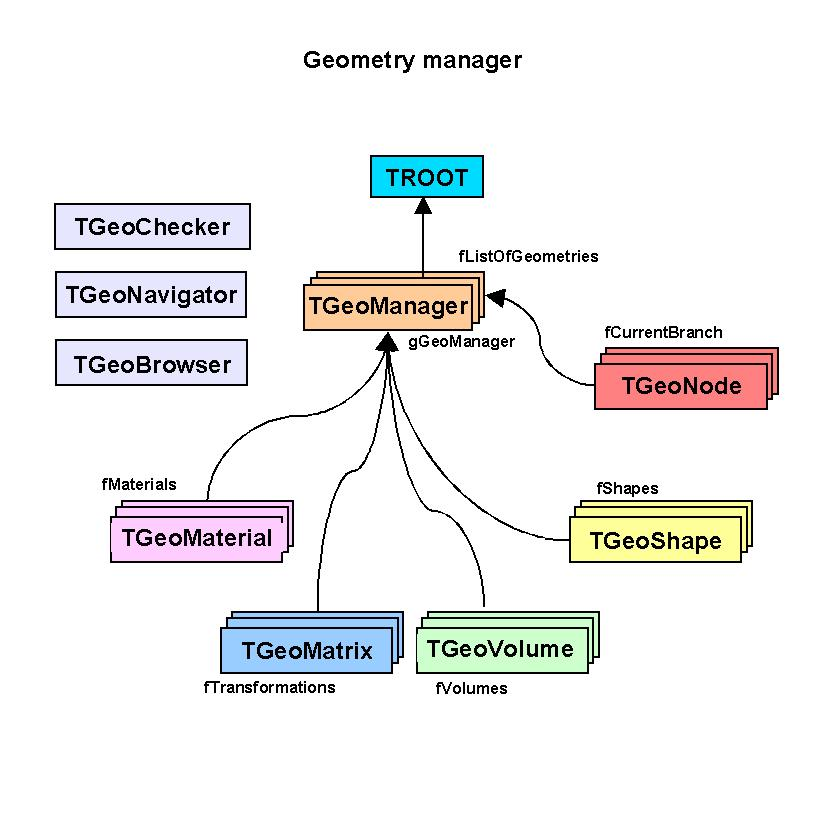*/
```
An interactive session
------------------------

Provided that a geometry was successfully built and closed (for instance the
previous example \$ROOTSYS/tutorials/rootgeom.C ), the manager class will register
itself to ROOT and the logical/physical structures will become immediately browsable.
The ROOT browser will display starting from the geometry folder : the list of
transformations and media, the top volume and the top logical node. These last
two can be fully expanded, any intermediate volume/node in the browser being subject
of direct access context menu operations (right mouse button click). All user
utilities of classes TGeoManager, TGeoVolume and TGeoNode can be called via the

```
/*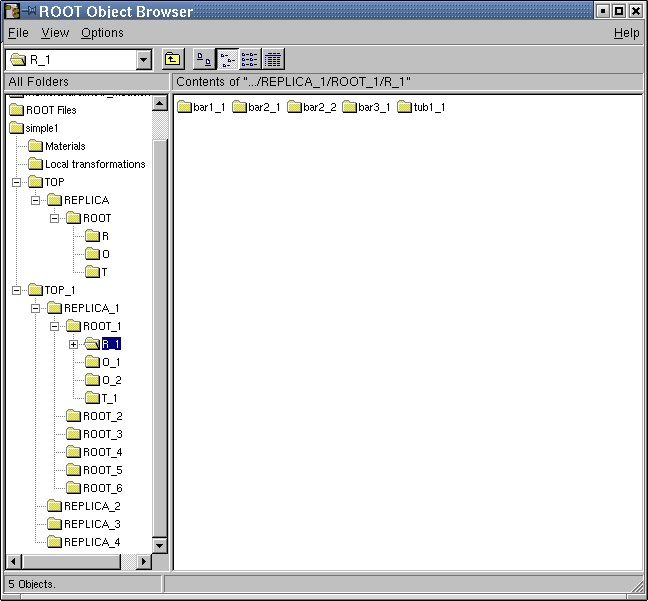*/
```
--- Drawing the geometry

Any logical volume can be drawn via TGeoVolume::Draw() member function.
This can be direcly accessed from the context menu of the volume object
directly from the browser.
There are several drawing options that can be set with
TGeoManager::SetVisOption(Int_t opt) method :
opt=0 - only the content of the volume is drawn, N levels down (default N=3).
This is the default behavior. The number of levels to be drawn can be changed
via TGeoManager::SetVisLevel(Int_t level) method.

```
/*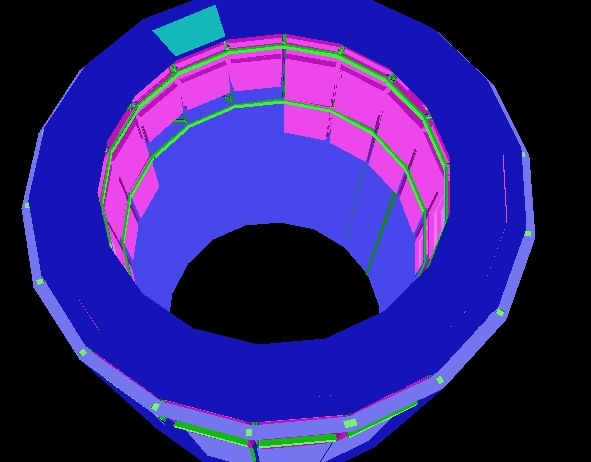*/
```
opt=1 - the final leaves (e.g. daughters with no containment) of the branch
starting from volume are drawn down to the current number of levels.
WARNING : This mode is memory consuming
depending of the size of geometry, so drawing from top level within this mode
should be handled with care for expensive geometries. In future there will be
a limitation on the maximum number of nodes to be visualized.

```
/*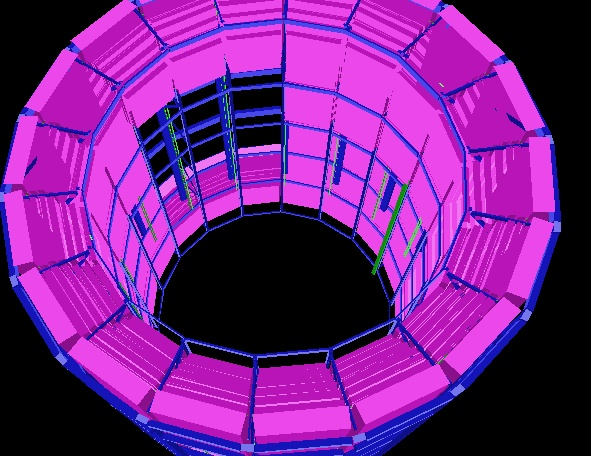*/
```
opt=2 - only the clicked volume is visualized. This is automatically set by
TGeoVolume::DrawOnly() method
opt=3 - only a given path is visualized. This is automatically set by
TGeoVolume::DrawPath(const char *path) method

The current view can be exploded in cartesian, cylindrical or spherical
coordinates :
TGeoManager::SetExplodedView(Int_t opt). Options may be :
- 0  - default (no bombing)
- 1  - cartesian coordinates. The bomb factor on each axis can be set with
TGeoManager::SetBombX(Double_t bomb) and corresponding Y and Z.
- 2  - bomb in cylindrical coordinates. Only the bomb factors on Z and R
are considered

```
/*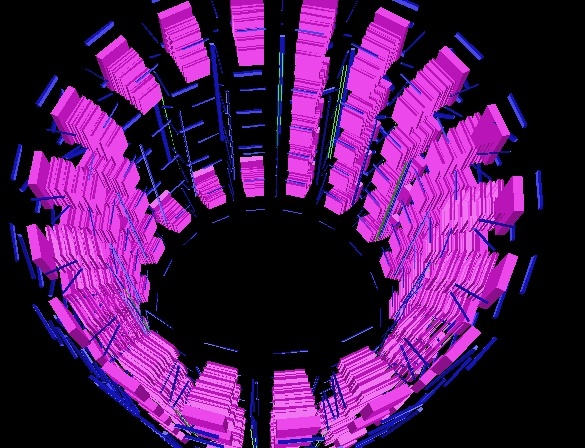*/
```
- 3  - bomb in radial spherical coordinate : TGeoManager::SetBombR()

Volumes themselves support different visualization settings :
- TGeoVolume::SetVisibility() : set volume visibility.
- TGeoVolume::VisibleDaughters() : set daughters visibility.
All these actions automatically updates the current view if any.

--- Checking the geometry

Several checking methods are accessible from the volume context menu. They
generally apply only to the visible parts of the drawn geometry in order to
ease geometry checking, and their implementation is in the TGeoChecker class
from the painting package.

1. Checking a given point.
Can be called from TGeoManager::CheckPoint(Double_t x, Double_t y, Double_t z).
This method is drawing the daughters of the volume containing the point one
level down, printing the path to the deepest physical node holding this point.
It also computes the closest distance to any boundary. The point will be drawn
in red.

```
/*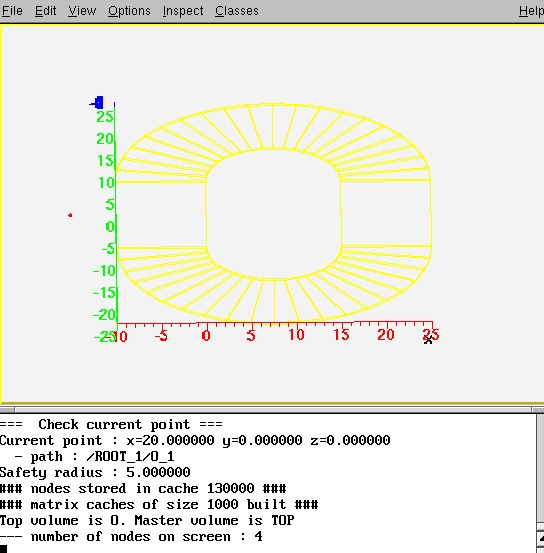*/
```
2. Shooting random points.
Can be called from TGeoVolume::RandomPoints() (context menu function) and
it will draw this volume with current visualization settings. Random points
are generated in the bounding box of the top drawn volume. The points are
classified and drawn with the color of their deepest container. Only points
in visible nodes will be drawn.

```
/*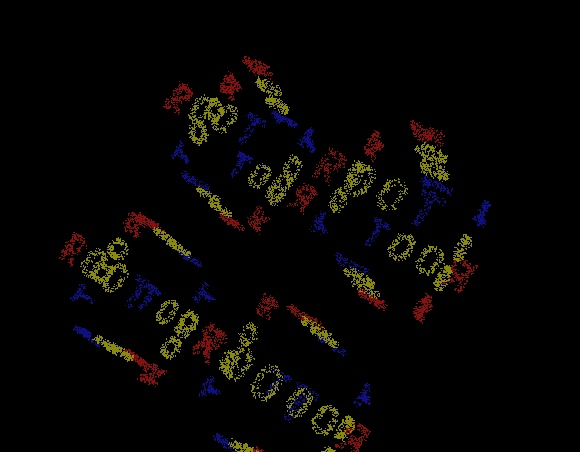*/
```

3. Raytracing.
Can be called from TGeoVolume::RandomRays() (context menu of volumes) and
will shoot rays from a given point in the local reference frame with random
directions. The intersections with displayed nodes will appear as segments
having the color of the touched node. Drawn geometry will be then made invisible
in order to enhance rays.

```
/*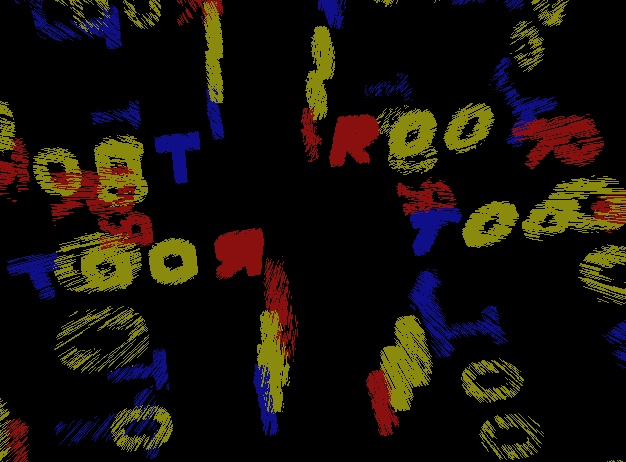*/

TGeoManager()
``` Default constructor.
```

TGeoManager(const char *name, const char *title) :TNamed(name, title)
``` Constructor.
```

void Init()
``` Initialize manager class.
```

~TGeoManager()
``` Destructor
```

``` Add a material to the list. Returns index of the material in list.
```

``` Add a matrix to the list. Returns index of the matrix in list.
```

``` Add a shape to the list. Returns index of the shape in list.
```

Int_t AddTrack(Int_t id, Int_t pdgcode, TObject *particle)
``` Add a track to the list of tracks
```

TVirtualGeoTrack* MakeTrack(Int_t id, Int_t pdgcode, TObject *particle)
``` Makes a primary track but do not attach it to the list of tracks. The track
can be attached as daughter to another one with TVirtualGeoTrack::AddTrack
```

``` Add a volume to the list. Returns index of the volume in list.
```

void Browse(TBrowser *b)
``` Describe how to browse this object.
```

void BombTranslation(const Double_t *tr, Double_t *bombtr)
``` Get the new 'bombed' translation vector according current exploded view mode.
```

void UnbombTranslation(const Double_t *tr, Double_t *bombtr)
``` Get the new 'unbombed' translation vector according current exploded view mode.
```

void BuildCache(Bool_t dummy, Bool_t nodeid)
``` Builds the cache for physical nodes and global matrices.
```

void BuildIdArray()
``` Builds node id array.
```

void RegisterMatrix(const TGeoMatrix *matrix)
``` Register a matrix to the list of matrices. It will be cleaned-up at the
destruction TGeoManager.
```

TGeoVolume* Division(const char *name, const char *mother, Int_t iaxis, Int_t ndiv, Double_t start, Double_t step, Int_t numed, Option_t *option)
``` Create a new volume by dividing an existing one (GEANT3 like)

Divides MOTHER into NDIV divisions called NAME
along axis IAXIS starting at coordinate value START
and having size STEP. The created volumes will have tracking
media ID=NUMED (if NUMED=0 -> same media as MOTHER)
The behavior of the division operation can be triggered using OPTION :
OPTION (case insensitive) :
N  - divide all range in NDIV cells (same effect as STEP<=0) (GSDVN in G3)
NX - divide range starting with START in NDIV cells          (GSDVN2 in G3)
S  - divide all range with given STEP. NDIV is computed and divisions will be centered
in full range (same effect as NDIV<=0)                (GSDVS, GSDVT in G3)
SX - same as DVS, but from START position.                   (GSDVS2, GSDVT2 in G3)
```

void Matrix(Int_t index, Double_t theta1, Double_t phi1, Double_t theta2, Double_t phi2, Double_t theta3, Double_t phi3)
``` Create rotation matrix named 'mat<index>'.

index    rotation matrix number
theta1   polar angle for axis X
phi1     azimuthal angle for axis X
theta2   polar angle for axis Y
phi2     azimuthal angle for axis Y
theta3   polar angle for axis Z
phi3     azimuthal angle for axis Z

```

TGeoMaterial* Material(const char *name, Double_t a, Double_t z, Double_t dens, Int_t uid,Double_t radlen, Double_t intlen)
``` Create material with given A, Z and density, having an unique id.
```

TGeoMaterial* Mixture(const char *name, Float_t *a, Float_t *z, Double_t dens, Int_t nelem, Float_t *wmat, Int_t uid)
``` Create mixture OR COMPOUND IMAT as composed by THE BASIC nelem
materials defined by arrays A,Z and WMAT, having an unique id.
```

TGeoMaterial* Mixture(const char *name, Double_t *a, Double_t *z, Double_t dens, Int_t nelem, Double_t *wmat, Int_t uid)
``` Create mixture OR COMPOUND IMAT as composed by THE BASIC nelem
materials defined by arrays A,Z and WMAT, having an unique id.
```

TGeoMedium* Medium(const char *name, Int_t numed, Int_t nmat, Int_t isvol, Int_t ifield, Double_t fieldm, Double_t tmaxfd, Double_t stemax, Double_t deemax, Double_t epsil, Double_t stmin)
``` Create tracking medium

numed      tracking medium number assigned
name      tracking medium name
nmat      material number
isvol     sensitive volume flag
ifield    magnetic field
fieldm    max. field value (kilogauss)
tmaxfd    max. angle due to field (deg/step)
stemax    max. step allowed
deemax    max. fraction of energy lost in a step
epsil     tracking precision (cm)
stmin     min. step due to continuous processes (cm)

ifield = 0 if no magnetic field; ifield = -1 if user decision in guswim;
ifield = 1 if tracking performed with g3rkuta; ifield = 2 if tracking
performed with g3helix; ifield = 3 if tracking performed with g3helx3.

```

void Node(const char *name, Int_t nr, const char *mother, Double_t x, Double_t y, Double_t z, Int_t irot, Bool_t isOnly, Float_t *upar, Int_t npar)
``` Create a node called <name_nr> pointing to the volume called <name>
as daughter of the volume called <mother> (gspos). The relative matrix is
made of : a translation (x,y,z) and a rotation matrix named <matIROT>.
In case npar>0, create the volume to be positioned in mother, according
its actual parameters (gsposp).
NAME   Volume name
NUMBER Copy number of the volume
MOTHER Mother volume name
X      X coord. of the volume in mother ref. sys.
Y      Y coord. of the volume in mother ref. sys.
Z      Z coord. of the volume in mother ref. sys.
IROT   Rotation matrix number w.r.t. mother ref. sys.
ISONLY ONLY/MANY flag
```

void Node(const char *name, Int_t nr, const char *mother, Double_t x, Double_t y, Double_t z, Int_t irot, Bool_t isOnly, Double_t *upar, Int_t npar)
``` Create a node called <name_nr> pointing to the volume called <name>
as daughter of the volume called <mother> (gspos). The relative matrix is
made of : a translation (x,y,z) and a rotation matrix named <matIROT>.
In case npar>0, create the volume to be positioned in mother, according
its actual parameters (gsposp).
NAME   Volume name
NUMBER Copy number of the volume
MOTHER Mother volume name
X      X coord. of the volume in mother ref. sys.
Y      Y coord. of the volume in mother ref. sys.
Z      Z coord. of the volume in mother ref. sys.
IROT   Rotation matrix number w.r.t. mother ref. sys.
ISONLY ONLY/MANY flag
```

TGeoVolume* Volume(const char *name, const char *shape, Int_t nmed, Float_t *upar, Int_t npar)
``` Create a volume in GEANT3 style.
NAME   Volume name
SHAPE  Volume type
NMED   Tracking medium number
NPAR   Number of shape parameters
UPAR   Vector containing shape parameters
```

TGeoVolume* Volume(const char *name, const char *shape, Int_t nmed, Double_t *upar, Int_t npar)
``` Create a volume in GEANT3 style.
NAME   Volume name
SHAPE  Volume type
NMED   Tracking medium number
NPAR   Number of shape parameters
UPAR   Vector containing shape parameters
```

void ClearAttributes()
``` Reset all attributes to default ones. Default attributes for visualization
are those defined before closing the geometry.
```

void CloseGeometry(Option_t *option)
``` Closing geometry implies checking the geometry validity, fixing shapes
with negative parameters (run-time shapes)building the cache manager,
voxelizing all volumes, counting the total number of physical nodes and
registring the manager class to the browser.
```

void ClearOverlaps()
``` Clear the list of overlaps.
```

void ClearShape(const TGeoShape *shape)
``` Remove a shape from the list of shapes.
```

void CleanGarbage()
``` Clean temporary volumes and shapes from garbage collection.
```

void CdNode(Int_t nodeid)
``` Change current path to point to the node having this id.
Node id has to be in range : 0 to fNNodes-1 (no check for performance reasons)
```

Int_t GetCurrentNodeId() const

void CdTop()
``` Make top level node the current node. Updates the cache accordingly.
Determine the overlapping state of current node.
```

void CdUp()
``` Go one level up in geometry. Updates cache accordingly.
Determine the overlapping state of current node.
```

void CdDown(Int_t index)
``` Make a daughter of current node current. Can be called only with a valid
daughter index (no check). Updates cache accordingly.
```

Bool_t cd(const char *path)
``` Browse the tree of nodes starting from fTopNode according to pathname.
Changes the path accordingly.
```

Int_t CountNodes(const TGeoVolume *vol, Int_t nlevels, Int_t option)
``` Count the total number of nodes starting from a volume, nlevels down.
```

void DefaultAngles()
``` Set default angles for a given view.
```

void DrawCurrentPoint(Int_t color)
``` Draw current point in the same view.
```

void AnimateTracks(Double_t tmin, Double_t tmax, Int_t nframes, Option_t *option)
``` Draw animation of tracks
```

void DrawTracks(Option_t *option)
``` Draw tracks over the geometry, according to option. By default, only
primaries are drawn. See TGeoTrack::Draw() for additional options.
```

void DrawPath(const char *path)
``` Draw current path
```

void RandomPoints(const TGeoVolume *vol, Int_t npoints, Option_t *option)
``` Draw random points in the bounding box of a volume.
```

void Test(Int_t npoints, Option_t *option)
``` Check time of finding "Where am I" for n points.
```

void TestOverlaps(const char* path)
``` Geometry overlap checker based on sampling.
```

void GetBranchNames(Int_t *names) const
``` Fill volume names of current branch into an array.
```

const char* GetPdgName(Int_t pdg) const
``` Get name for given pdg code;
```

void SetPdgName(Int_t pdg, const char *name)

void GetBranchNumbers(Int_t *copyNumbers, Int_t *volumeNumbers) const
``` Fill node copy numbers of current branch into an array.
```

void GetBranchOnlys(Int_t *isonly) const
``` Fill node copy numbers of current branch into an array.
```

void GetBombFactors(Double_t &bombx, Double_t &bomby, Double_t &bombz, Double_t &bombr) const
``` Retrieve cartesian and radial bomb factors.
```

TGeoHMatrix* GetHMatrix()

Int_t GetVisLevel() const
``` Returns current depth to which geometry is drawn.
```

Int_t GetVisOption() const
``` Returns current depth to which geometry is drawn.
```

Int_t GetVirtualLevel()
``` Find level of virtuality of current overlapping node (number of levels
up having the same tracking media.
```

TVirtualGeoTrack* GetTrackOfId(Int_t id) const
``` Get track with a given ID.
```

TVirtualGeoTrack* GetParentTrackOfId(Int_t id) const
``` Get parent track with a given ID.
```

Int_t GetTrackIndex(Int_t id) const
``` Get index for track id, -1 if not found.
```

Bool_t GotoSafeLevel()
``` Go upwards the tree until a non-overlaping node
```

Int_t GetSafeLevel() const
``` Go upwards the tree until a non-overlaping node
```

TGeoNode* FindInCluster(Int_t *cluster, Int_t nc)
``` Find a node inside a cluster of overlapping nodes. Current node must
be on top of all the nodes in cluster. Always nc>1.
```

Int_t GetTouchedCluster(Int_t start, Double_t *point, Int_t *check_list, Int_t ncheck, Int_t *result)
``` Make the cluster of overlapping nodes in a voxel, containing point in reference
of the mother. Returns number of nodes containing the point. Nodes should not be
offsets.
```

void DefaultColors()
``` Set default volume colors according to A of material
```

Double_t Safety(Bool_t inside)
``` Compute safe distance from the current point. This represent the distance
from POINT to the closest boundary.
```

void SafetyOverlaps()
``` Compute safe distance from the current point within an overlapping node
```

void SetVolumeAttribute(const char *name, const char *att, Int_t val)
``` Set volume attributes in G3 style.
```

void SetBombFactors(Double_t bombx, Double_t bomby, Double_t bombz, Double_t bombr)
``` Set factors that will "bomb" all translations in cartesian and cylindrical coordinates.
```

void SetClippingShape(TGeoShape *shape)
``` Set a user-defined shape as clipping for ray tracing.
```

void SetMaxVisNodes(Int_t maxnodes)
``` set the maximum number of visible nodes.
```

void SetTopVisible(Bool_t vis)
``` make top volume visible on screen
```

void SetVisOption(Int_t option)
``` set drawing mode :
option=0 (default) all nodes drawn down to vislevel
option=1           leaves and nodes at vislevel drawn
option=2           path is drawn
```

void ViewLeaves(Bool_t flag)
``` Set visualization option (leaves only OR all volumes)
```

void SetVisDensity(Double_t density)
``` Set density threshold. Volumes with densities lower than this become
transparent.
```

void SetVisLevel(Int_t level)
``` set default level down to which visualization is performed
```

void SortOverlaps()
``` Sort overlaps by decreasing overlap distance. Extrusions comes first.
```

void OptimizeVoxels(const char *filename)
``` Optimize voxelization type for all volumes. Save best choice in a macro.
```

Int_t Parse(const char *expr, TString &expr1, TString &expr2, TString &expr3)
``` Parse a string boolean expression and do a syntax check. Find top
level boolean operator and returns its type. Fill the two
substrings to which this operator applies. The returned integer is :
-1 : parse error
0 : no boolean operator
1 : union - represented as '+' in expression
2 : difference (subtraction) - represented as '-' in expression
3 : intersection - represented as '*' in expression.
Paranthesys should be used to avoid ambiguites. For instance :
A+B-C will be interpreted as (A+B)-C which is not the same as A+(B-C)
eliminate not needed paranthesys
```

void SaveAttributes(const char *filename)
``` Save current attributes in a macro
```

TGeoNode* SearchNode(Bool_t downwards, const TGeoNode *skipnode)
``` Returns the deepest node containing fPoint, which must be set a priori.
```

TGeoNode* FindNextBoundary(Double_t stepmax, const char *path)
``` Find distance to next boundary and store it in fStep. Returns node to which this
boundary belongs. If PATH is specified, compute only distance to the node to which
PATH points. If STEPMAX is specified, compute distance only in case fSafety is smaller
than this value. STEPMAX represent the step to be made imposed by other reasons than
geometry (usually physics processes). Therefore in this case this method provides the
answer to the question : "Is STEPMAX a safe step ?" returning a NULL node and filling
fStep with a big number.
```

TGeoNode* FindNode(Bool_t safe_start)
``` Returns deepest node containing current point.
```

TGeoNode* FindNode(Double_t x, Double_t y, Double_t z)
``` Returns deepest node containing current point.
```

Double_t* FindNormalFast()
``` Computes fast normal to next crossed boundary, assuming that the current point
is close enough to the boundary. Works only after calling FindNextBoundary.
```

Double_t* FindNormal(Bool_t forward)
``` Computes normal vector to the next surface that will be or was already
crossed when propagating on a straight line from a given point/direction.
Returns the normal vector cosines in the MASTER coordinate system. The dot
product of the normal and the current direction is positive defined.
printf("Current node: %s forward=%i\n", GetPath(), forward);
```

Bool_t IsSameLocation(Double_t x, Double_t y, Double_t z, Bool_t change)
``` Checks if point (x,y,z) is still in the current node.
check if this is an overlapping node
```

Bool_t IsSamePoint(Double_t x, Double_t y, Double_t z) const

Bool_t IsInPhiRange() const
``` True if current node is in phi range
```

TGeoNode* InitTrack(Double_t *point, Double_t *dir)
``` Initialize current point and current direction vector (normalized)
in MARS. Return corresponding node.
```

TGeoNode* InitTrack(Double_t x, Double_t y, Double_t z, Double_t nx, Double_t ny, Double_t nz)
``` Initialize current point and current direction vector (normalized)
in MARS. Return corresponding node.
```

const char* GetPath() const
``` Get path to the current node in the form /node0/node1/...
```

Int_t GetByteCount(Option_t * /*option*/)
``` Get total size of geometry in bytes.
```

TVirtualGeoPainter* GetGeomPainter()
``` Make a default painter if none present. Returns pointer to it.
```

TGeoVolume* GetVolume(const char *name) const
``` Search for a named volume.
```

Int_t GetUID(const char *volname) const
``` Retreive unique id for a volume name. Return -1 if name not found.
```

TGeoMaterial* FindDuplicateMaterial(const TGeoMaterial *mat) const
``` Find if a given material duplicates an existing one.
```

TGeoMaterial* GetMaterial(const char *matname) const
``` Search for a named material.
```

TGeoMedium* GetMedium(const char *medium) const
``` Search for a named tracking medium.
```

TGeoMedium* GetMedium(Int_t numed) const
``` Search for a tracking medium with a given ID.
```

TGeoMaterial* GetMaterial(Int_t id) const
``` Return material at position id.
```

Int_t GetMaterialIndex(const char *matname) const
``` Return index of named material.
```

void RandomRays(Int_t nrays, Double_t startx, Double_t starty, Double_t startz)
``` Randomly shoot nrays and plot intersections with surfaces for current
top node.
```

void RemoveMaterial(Int_t index)
``` Remove material at given index.
```

void ResetUserData()
``` Sets all pointers TGeoVolume::fField to NULL. User data becomes decoupled
from geometry. Deletion has to be managed by users.
```

void RestoreMasterVolume()
``` Restore the master volume of the geometry.
```

void Voxelize(Option_t *option)
``` Voxelize all non-divided volumes.
```

``` Send "Modified" signal to painter.
```

TGeoVolume* MakeArb8(const char *name, const TGeoMedium *medium, Double_t dz, Double_t *vertices)
``` Make an TGeoArb8 volume.
```

TGeoVolume* MakeBox(const char *name, const TGeoMedium *medium, Double_t dx, Double_t dy, Double_t dz)
``` Make in one step a volume pointing to a box shape with given medium.
```

TGeoVolume* MakePara(const char *name, const TGeoMedium *medium, Double_t dx, Double_t dy, Double_t dz, Double_t alpha, Double_t theta, Double_t phi)
``` Make in one step a volume pointing to a paralelipiped shape with given medium.
```

TGeoVolume* MakeSphere(const char *name, const TGeoMedium *medium, Double_t rmin, Double_t rmax, Double_t themin, Double_t themax, Double_t phimin, Double_t phimax)
``` Make in one step a volume pointing to a sphere shape with given medium
```

TGeoVolume* MakeTorus(const char *name, const TGeoMedium *medium, Double_t r, Double_t rmin, Double_t rmax, Double_t phi1, Double_t dphi)
``` Make in one step a volume pointing to a torus shape with given medium.
```

TGeoVolume* MakeTube(const char *name, const TGeoMedium *medium, Double_t rmin, Double_t rmax, Double_t dz)
``` Make in one step a volume pointing to a tube shape with given medium.
```

TGeoVolume* MakeTubs(const char *name, const TGeoMedium *medium, Double_t rmin, Double_t rmax, Double_t dz, Double_t phi1, Double_t phi2)
``` Make in one step a volume pointing to a tube segment shape with given medium.
```

TGeoVolume* MakeEltu(const char *name, const TGeoMedium *medium, Double_t a, Double_t b, Double_t dz)
``` Make in one step a volume pointing to a tube shape with given medium
```

TGeoVolume* MakeHype(const char *name, const TGeoMedium *medium, Double_t rin, Double_t stin, Double_t rout, Double_t stout, Double_t dz)
``` Make in one step a volume pointing to a tube shape with given medium
```

TGeoVolume* MakeParaboloid(const char *name, const TGeoMedium *medium, Double_t rlo, Double_t rhi, Double_t dz)
``` Make in one step a volume pointing to a tube shape with given medium
```

TGeoVolume* MakeCtub(const char *name, const TGeoMedium *medium, Double_t rmin, Double_t rmax, Double_t dz, Double_t phi1, Double_t phi2, Double_t lx, Double_t ly, Double_t lz, Double_t tx, Double_t ty, Double_t tz)
``` Make in one step a volume pointing to a tube segment shape with given medium
```

TGeoVolume* MakeCone(const char *name, const TGeoMedium *medium, Double_t dz, Double_t rmin1, Double_t rmax1, Double_t rmin2, Double_t rmax2)
``` Make in one step a volume pointing to a cone shape with given medium.
```

TGeoVolume* MakeCons(const char *name, const TGeoMedium *medium, Double_t dz, Double_t rmin1, Double_t rmax1, Double_t rmin2, Double_t rmax2, Double_t phi1, Double_t phi2)
``` Make in one step a volume pointing to a cone segment shape with given medium
```

TGeoVolume* MakePcon(const char *name, const TGeoMedium *medium, Double_t phi, Double_t dphi, Int_t nz)
``` Make in one step a volume pointing to a polycone shape with given medium.
```

TGeoVolume* MakePgon(const char *name, const TGeoMedium *medium, Double_t phi, Double_t dphi, Int_t nedges, Int_t nz)
``` Make in one step a volume pointing to a polygone shape with given medium.
```

TGeoVolume* MakeTrd1(const char *name, const TGeoMedium *medium, Double_t dx1, Double_t dx2, Double_t dy, Double_t dz)
``` Make in one step a volume pointing to a TGeoTrd1 shape with given medium.
```

TGeoVolume* MakeTrd2(const char *name, const TGeoMedium *medium, Double_t dx1, Double_t dx2, Double_t dy1, Double_t dy2, Double_t dz)
``` Make in one step a volume pointing to a TGeoTrd2 shape with given medium.
```

TGeoVolume* MakeTrap(const char *name, const TGeoMedium *medium, Double_t dz, Double_t theta, Double_t phi, Double_t h1, Double_t bl1, Double_t tl1, Double_t alpha1, Double_t h2, Double_t bl2, Double_t tl2, Double_t alpha2)
``` Make in one step a volume pointing to a trapezoid shape with given medium.
```

TGeoVolume* MakeGtra(const char *name, const TGeoMedium *medium, Double_t dz, Double_t theta, Double_t phi, Double_t twist, Double_t h1, Double_t bl1, Double_t tl1, Double_t alpha1, Double_t h2, Double_t bl2, Double_t tl2, Double_t alpha2)
``` Make in one step a volume pointing to a twisted trapezoid shape with given medium.
```

TGeoVolume* MakeXtru(const char *name, const TGeoMedium *medium, Int_t nz)
``` Make a TGeoXtru-shaped volume with nz planes
```

TGeoPhysicalNode* MakePhysicalNode(const char *path)
``` Makes a physical node corresponding to a path. If PATH is not specified,
makes physical node matching current modeller state.
```

void ClearPhysicalNodes(Bool_t mustdelete)
``` Clear the current list of physical nodes, so that we can start over with a new list.
If MUSTDELETE is true, delete previous nodes.
```

TGeoVolumeAssembly* MakeVolumeAssembly(const char *name)
``` Make an assembly of volumes.
```

TGeoVolumeMulti* MakeVolumeMulti(const char *name, const TGeoMedium *medium)
``` Make a TGeoVolumeMulti handling a list of volumes.
```

void SetExplodedView(Int_t ibomb)
``` Set type of exploding view (see TGeoPainter::SetExplodedView())
```

void SetPhiRange(Double_t phimin, Double_t phimax)
``` Set cut phi range
```

void SetNsegments(Int_t nseg)
``` Set number of segments for approximating circles in drawing.
```

Int_t GetNsegments() const
``` Get number of segments approximating circles
```

void BuildDefaultMaterials()
``` Build the default materials. A list of those can be found in ...
new TGeoMaterial("Air", 14.61, 7.3, 0.001205);
```

TGeoNode* Step(Bool_t is_geom, Bool_t cross)
``` Make a rectiliniar step of length fStep from current point (fPoint) on current
direction (fDirection). If the step is imposed by geometry, is_geom flag
must be true (default). The cross flag specifies if the boundary should be
crossed in case of a geometry step (default true). Returns new node after step.
Set also on boundary condition.
```

TGeoNode* SamplePoints(Int_t npoints, Double_t &dist, Double_t epsil, const char* g3path)
``` shoot npoints randomly in a box of 1E-5 arround current point.
return minimum distance to points outside
```

void SetTopVolume(TGeoVolume *vol)
``` Set the top volume and corresponding node as starting point of the geometry.
```

void SelectTrackingMedia()
``` Define different tracking media.
```

void CheckPoint(Double_t x, Double_t y, Double_t z, Option_t *option)
``` Classify a given point. See TGeoChecker::CheckPoint().
```

void CheckGeometry(Option_t * /*option*/)
``` Instanciate a TGeoChecker object and investigates the geometry according to
option. Not implemented yet.
check shapes first
```

void CheckOverlaps(Double_t ovlp, Option_t * option)
``` Check all geometry for illegal overlaps within a limit OVLP.
```

void PrintOverlaps() const
``` Prints the current list of overlaps.
```

void UpdateCurrentPosition(Double_t * /*nextpoint*/)
``` Computes and changes the current node according to the new position.
Not implemented.
```

Double_t Weight(Double_t precision, Option_t *option)
``` Estimate weight of volume VOL with a precision SIGMA(W)/W better than PRECISION.
Option can be "v" - verbose (default)
```

ULong_t SizeOf(const TGeoNode * /*node*/, Option_t * /*option*/)
``` computes the total size in bytes of the branch starting with node.
The option can specify if all the branch has to be parsed or only the node
```

void Streamer(TBuffer &R__b)
``` Stream an object of class TGeoManager.
```

Int_t Export(const char *filename, const char *name, Option_t *option)
``` Export this geometry on filename with a key=name
By default the geometry is saved without the voxelisation info.
Use option 'v" to save the voxelisation info.
```

TGeoManager* Import(const char *filename, const char *name, Option_t * /*option*/)
```static function
Import in memory from filename the geometry with key=name.
if name="" (default), the first TGeoManager object in the file is returned.
Note that this function deletes the current gGeoManager (if one)
before importing the new object.
```

Int_t* GetIntBuffer(Int_t length)
``` Get a temporary buffer of Int_t*
```

Double_t* GetDblBuffer(Int_t length)
``` Get a temporary buffer of Double_t*
```

Bool_t GetTminTmax(Double_t &tmin, Double_t &tmax) const
``` Get time cut for drawing tracks.
```

void SetTminTmax(Double_t tmin, Double_t tmax)
``` Set time cut interval for drawing tracks. If called with no arguments, time
cut will be disabled.
```

void MasterToTop(const Double_t *master, Double_t *top) const
``` Convert coordinates from master volume frame to top.
```

void TopToMaster(const Double_t *top, Double_t *master) const
``` Convert coordinates from top volume frame to master.
```

### Inline Functions

```                     Bool_t IsLoopingVolumes() const
void SetLoopVolumes(Bool_t flag = kTRUE)
Bool_t IsFolder() const
TGeoShape* GetClippingShape() const
TVirtualGeoPainter* GetPainter() const
Int_t GetBombMode() const
Int_t GetMaxVisNodes() const
Double_t GetTmax() const
TGeoVolume* GetPaintVolume() const
Double_t GetVisDensity() const
Bool_t IsDrawingExtra() const
Bool_t IsVisLeaves() const
void SetClipping(Bool_t flag = kTRUE)
void SetPaintVolume(TGeoVolume* vol)
void SetDrawExtraPaths(Bool_t flag = kTRUE)
void SetParticleName(const char* pname)
const char* GetParticleName() const
Bool_t IsClosed() const
void SetCurrentTrack(Int_t i)
void SetCurrentTrack(TVirtualGeoTrack* track)
Int_t GetNtracks() const
TVirtualGeoTrack* GetCurrentTrack()
TVirtualGeoTrack* GetLastTrack()
const Double_t* GetLastPoint() const
TVirtualGeoTrack* GetTrack(Int_t index)
Double_t GetSafeDistance() const
Double_t GetStep() const
Bool_t IsAnimatingTracks() const
Bool_t IsCheckingOverlaps() const
Bool_t IsMatrixTransform() const
Bool_t IsMatrixReflection() const
Bool_t IsSameLocation() const
Bool_t IsStartSafe() const
void SetStartSafe(Bool_t flag = kTRUE)
void SetMatrixTransform(Bool_t on = kTRUE)
void SetMatrixReflection(Bool_t flag = kTRUE)
void SetStep(Double_t step)
Bool_t IsCurrentOverlapping() const
Bool_t IsEntering() const
Bool_t IsExiting() const
Bool_t IsStepEntering() const
Bool_t IsStepExiting() const
Bool_t IsOutside() const
Bool_t IsOnBoundary() const
Bool_t IsNullStep() const
void SetOutside(Bool_t flag = kTRUE)
void ClearTracks()
UChar_t* GetBits()
Bool_t IsStreamingVoxels() const
TObjArray* GetListOfNodes()
TObjArray* GetListOfPhysicalNodes()
TObjArray* GetListOfOverlaps()
TObjArray* GetListOfMatrices() const
TList* GetListOfMaterials() const
TList* GetListOfMedia() const
TObjArray* GetListOfVolumes() const
TObjArray* GetListOfGVolumes() const
TObjArray* GetListOfShapes() const
TObjArray* GetListOfUVolumes() const
TObjArray* GetListOfTracks() const
TGeoNode* GetNode(Int_t level) const
Int_t GetNodeId() const
TGeoNode* GetNextNode() const
TGeoNode* GetMother(Int_t up = 1) const
TGeoHMatrix* GetCurrentMatrix() const
TGeoHMatrix* GetGLMatrix() const
TGeoNode* GetCurrentNode() const
Double_t* GetCurrentPoint() const
Double_t* GetCurrentDirection() const
TGeoVolume* GetCurrentVolume() const
Double_t* GetCldirChecked() const
Double_t* GetCldir() const
const Double_t* GetNormal() const
Int_t GetLevel() const
Int_t GetStackLevel() const
TGeoVolume* GetMasterVolume() const
TGeoVolume* GetTopVolume() const
TGeoNode* GetTopNode() const
TGeoPhysicalNode* GetPhysicalNode(Int_t i) const
void SetCurrentPoint(Double_t* point)
void SetCurrentPoint(Double_t x, Double_t y, Double_t z)
void SetLastPoint(Double_t x, Double_t y, Double_t z)
void SetCurrentDirection(Double_t* dir)
void SetCurrentDirection(Double_t nx, Double_t ny, Double_t nz)
void SetCldirChecked(Double_t* dir)
void LocalToMaster(const Double_t* local, Double_t* master) const
void LocalToMasterVect(const Double_t* local, Double_t* master) const
void LocalToMasterBomb(const Double_t* local, Double_t* master) const
void MasterToLocal(const Double_t* master, Double_t* local) const
void MasterToLocalVect(const Double_t* master, Double_t* local) const
void MasterToLocalBomb(const Double_t* master, Double_t* local) const
TGeoVolume* GetVolume(Int_t uid) const
Int_t GetNNodes()
TGeoNodeCache* GetCache() const
void SetCache(const TGeoNodeCache* cache)
void SetAnimateTracks(Bool_t flag = kTRUE)
Int_t PushPath(Int_t startlevel = 0)
Bool_t PopPath()
Bool_t PopPath(Int_t index)
Int_t PushPoint(Int_t startlevel = 0)
Bool_t PopPoint()
Bool_t PopPoint(Int_t index)
void PopDummy(Int_t ipop = 9999)
TClass* Class()
TClass* IsA() const
void ShowMembers(TMemberInspector& insp, char* parent)
void StreamerNVirtual(TBuffer& b)
TGeoManager TGeoManager(const TGeoManager&)
TGeoManager& operator=(const TGeoManager&)
```

Author: Andrei Gheata 25/10/01
Last update: root/geom:\$Name: \$:\$Id: TGeoManager.cxx,v 1.105 2004/12/14 19:46:53 brun Exp \$